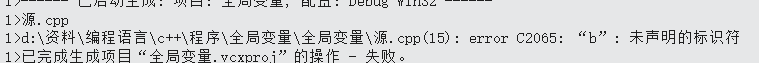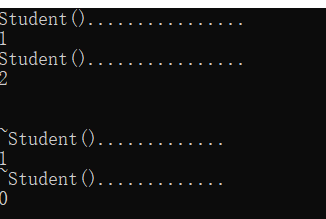# 3、静态变量和函数

### 1、全局变量

#include<iostream>
using namespace std;

int a = 1;

void func(int &a)
{
a++;                         //自加运算
cout << a << endl;           //打印a的值
}

int main()
{
func(a);                     //调用
func(a);
func(a);
return 0;
}

注意：在声明函数是，一定要加上 ‘&’，否则a的值不会变化。 或者用指针

### 2、局部变量

#include<iostream>
using namespace std;

int main()
{

int a;
a = 4;
cout << a << endl;
{
int b = 1;
cout << b << endl;
}
cout << b << endl;
return 0;
}#include<iostream>
using namespace std;

void func()
{
cout << a << endl;
}

int main()
{

int a;
a = 4;
func();
return 0;
}

### 3、静态变量

#include<iostream>
using namespace std;

static int a = 1;

void func(int &a)
{
a++;                         //自加运算
cout << a << endl;           //打印a的值
}

int main()
{
func(a);                     //调用
func(a);
func(a);
return 0;
}

### 4、静态变量在c++中应用

#include<iostream>
#include<string>
using namespace std;

class Student
{
public:
Student(string name, int age, float store,int count);              //声明构造函数
~Student();                                              //声明析构函数
int GetCount();
private:
string name;
int age;
float store;
int count;                                           //记录学生数量

};
Student::Student(string name, int age, float store,int count)
{
this->name = name;
this->age = age;
this->store = store;
this->count = count;
cout << "Student()................" << endl;
}
Student::~Student()
{
cout << "~Student()............." << endl;
}
int Student::GetCount()
{
return this->count;
}
int main()
{
Student stu1("jiang", 18, 99.3,1);
cout << stu1.GetCount() << endl;
Student stu2("wang", 19, 100,2);
cout << stu2.GetCount() << endl;
cout << endl << endl;

return 0;
}

所以，在调用构造函数是，count++，在调用析构函数是，count--；

还要注意的是需要count变量不会因为stu1的释放而消失，即count是属于student这个类的成员数据

#include<iostream>
#include<string>
using namespace std;

class Student
{
public:
Student(string name, int age, float store);              //声明构造函数
~Student();                                              //声明析构函数
int GetCount();
private:
string name;
int age;
float store;
static int count;                                        //定义静态数据成员变量

};
Student::Student(string name, int age, float store)
{
this->name = name;
this->age = age;
this->store = store;
count++;                                                  //count++
cout << "Student()................" << endl;
}
Student::~Student()
{
count--;                                                 //count--
cout << "~Student()............." << endl;
}
int Student::GetCount()
{
return this->count;
}
int Student::count = 0;
int main()
{
Student *stu1=new Student("jiang", 18, 99.3);          //用指针来声明，方便之后挨个释放是观察count的值
cout << stu1->GetCount() << endl;
Student *stu2=new Student("wang", 19, 100);
cout << stu2->GetCount() << endl;
cout << endl << endl;

delete stu1;
cout << stu2->GetCount() << endl;
delete stu2;
cout << Student::GetCount() << endl;

return 0;
}分析：在程序中，每定义一个stu，count加1；而dlete只后，count减1；

a、由于静态程序是属于类的，即不能根据对象来定义它；需要在主函数外定义

int Student::count = 0;

b、可以通过类名来访问它

cout << Student::count << endl;

### 6、静态变量与动态变量的区别

a、静态变量通常是在变量定义时就分定存储单元并一直保持不变，直至整个程序结束。静态变量，全局动态变量都是静态存储。

b、动态变量是在程序执行过程中，使用它时才分配存储单元，使用完毕立即释放。

c、静态变量只会初始化一次，重复初始化会出现语法错误。如果静态变量没有初始化，则系统自动定义为0，而动态变量为随机值。

d、全局数据区：static 数据, 全局变量, const常量。

堆区：由程序员自己new出来的动态数据， 需要手动释放。若忘记释放，会造成内存泄漏，则程序结束时会由操作系统回收。

栈区：函数内部变量，由IDE自动分配，结束时自动释放。

posted @ 2019-09-28 23:55  aakihi  阅读(300)  评论(0编辑  收藏  举报